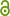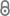Dequantizing the Quantum Singular Value Transformation: Hardness and Applications to Quantum Chemistry and the Quantum PCP Conjecture

S. Gharibian, F.L. Gall, ArXiv:2111.09079 (2021).https://arxiv.org/abs/2111.09079
Preprint | English
Author
Abstract
The Quantum Singular Value Transformation (QSVT) is a recent technique that gives a unified framework to describe most quantum algorithms discovered so far, and may lead to the development of novel quantum algorithms. In this paper we investigate the hardness of classically simulating the QSVT. A recent result by Chia, Gily\'en, Li, Lin, Tang and Wang (STOC 2020) showed that the QSVT can be efficiently "dequantized" for low-rank matrices, and discussed its implication to quantum machine learning. In this work, motivated by establishing the superiority of quantum algorithms for quantum chemistry and making progress on the quantum PCP conjecture, we focus on the other main class of matrices considered in applications of the QSVT, sparse matrices. We first show how to efficiently "dequantize", with arbitrarily small constant precision, the QSVT associated with a low-degree polynomial. We apply this technique to design classical algorithms that estimate, with constant precision, the singular values of a sparse matrix. We show in particular that a central computational problem considered by quantum algorithms for quantum chemistry (estimating the ground state energy of a local Hamiltonian when given, as an additional input, a state sufficiently close to the ground state) can be solved efficiently with constant precision on a classical computer. As a complementary result, we prove that with inverse-polynomial precision, the same problem becomes BQP-complete. This gives theoretical evidence for the superiority of quantum algorithms for chemistry, and strongly suggests that said superiority stems from the improved precision achievable in the quantum setting. We also discuss how this dequantization technique may help make progress on the central quantum PCP conjecture.
Publishing Year
Journal Title
arXiv:2111.09079
LibreCat-ID

Cite this

Gharibian S, Gall FL. Dequantizing the Quantum Singular Value Transformation: Hardness and  Applications to Quantum Chemistry and the Quantum PCP Conjecture. arXiv:211109079. Published online 2021.
Gharibian, S., & Gall, F. L. (2021). Dequantizing the Quantum Singular Value Transformation: Hardness and  Applications to Quantum Chemistry and the Quantum PCP Conjecture. In arXiv:2111.09079.
@article{Gharibian_Gall_2021, title={Dequantizing the Quantum Singular Value Transformation: Hardness and  Applications to Quantum Chemistry and the Quantum PCP Conjecture}, journal={arXiv:2111.09079}, author={Gharibian, Sevag and Gall, François Le}, year={2021} }
Gharibian, Sevag, and François Le Gall. “Dequantizing the Quantum Singular Value Transformation: Hardness and  Applications to Quantum Chemistry and the Quantum PCP Conjecture.” ArXiv:2111.09079, 2021.
S. Gharibian and F. L. Gall, “Dequantizing the Quantum Singular Value Transformation: Hardness and  Applications to Quantum Chemistry and the Quantum PCP Conjecture,” arXiv:2111.09079. 2021.
Gharibian, Sevag, and François Le Gall. “Dequantizing the Quantum Singular Value Transformation: Hardness and  Applications to Quantum Chemistry and the Quantum PCP Conjecture.” ArXiv:2111.09079, 2021.
All files available under the following license(s):Closed Access